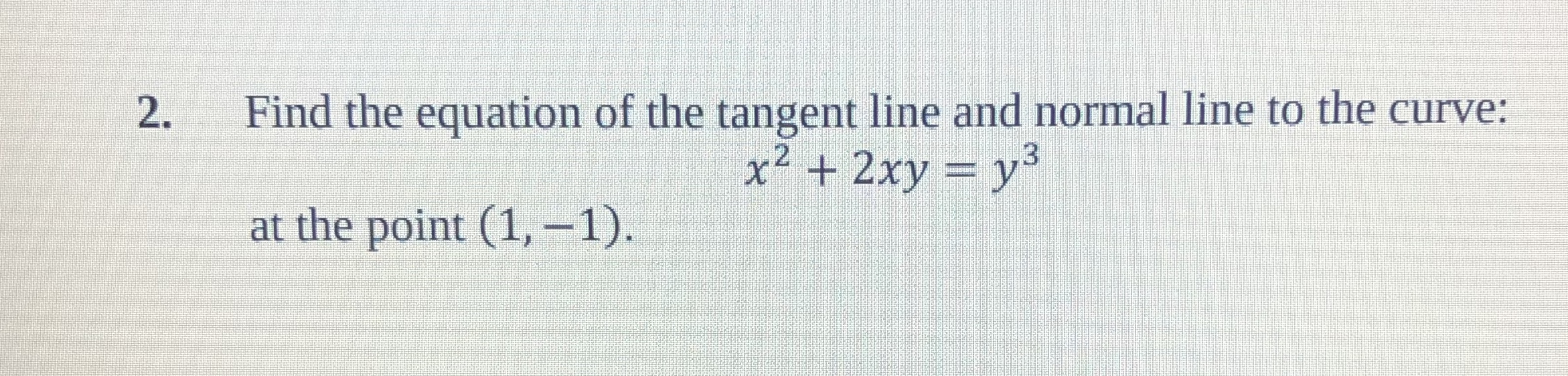# Find the equation of the tangent line and normal line to the curve:2.x2 + 2xy = y3at the point (1,-1)

Question
69 viewshelp_outlineImage TranscriptioncloseFind the equation of the tangent line and normal line to the curve: 2. x2 + 2xy = y3 at the point (1,-1) fullscreen
check_circle

Step 1

Firstly, we find  dy/dx by differentiating both sides with respect to x

Step 2

We know that slope of tangent(m1) to curve at point (x1,y1) is the value of dy/dx at (x1,y1) and the we find the equation of tangent at (x1,y1) by using formula shown on board.

Step 3

Now we know that tangent and normal are perpendicular, So the product of their slopes is -1,Since, slope of tan...

### Want to see the full answer?

See Solution

#### Want to see this answer and more?

Solutions are written by subject experts who are available 24/7. Questions are typically answered within 1 hour.*

See Solution
*Response times may vary by subject and question.
Tagged in

### Derivative